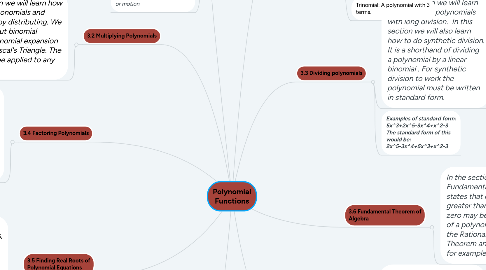# Polynomial FunctionsDacia Harrison
Get Started. It's FreePolynomial Functions## 8. 3.1 Polynomials

### 8.3. Degree of a monomial: Is the sum of the exponents of the variables.

8.3.1. Ex: 4ab^2b^1, the degree would be 3.

### 8.4. Degree of a polynomials: Is the term with the highest degree.

8.4.1. Ex: 5x^3+6x^2+4x, the degree would be 3 because it is the highest degree plus it is attached to the leading coefficient.